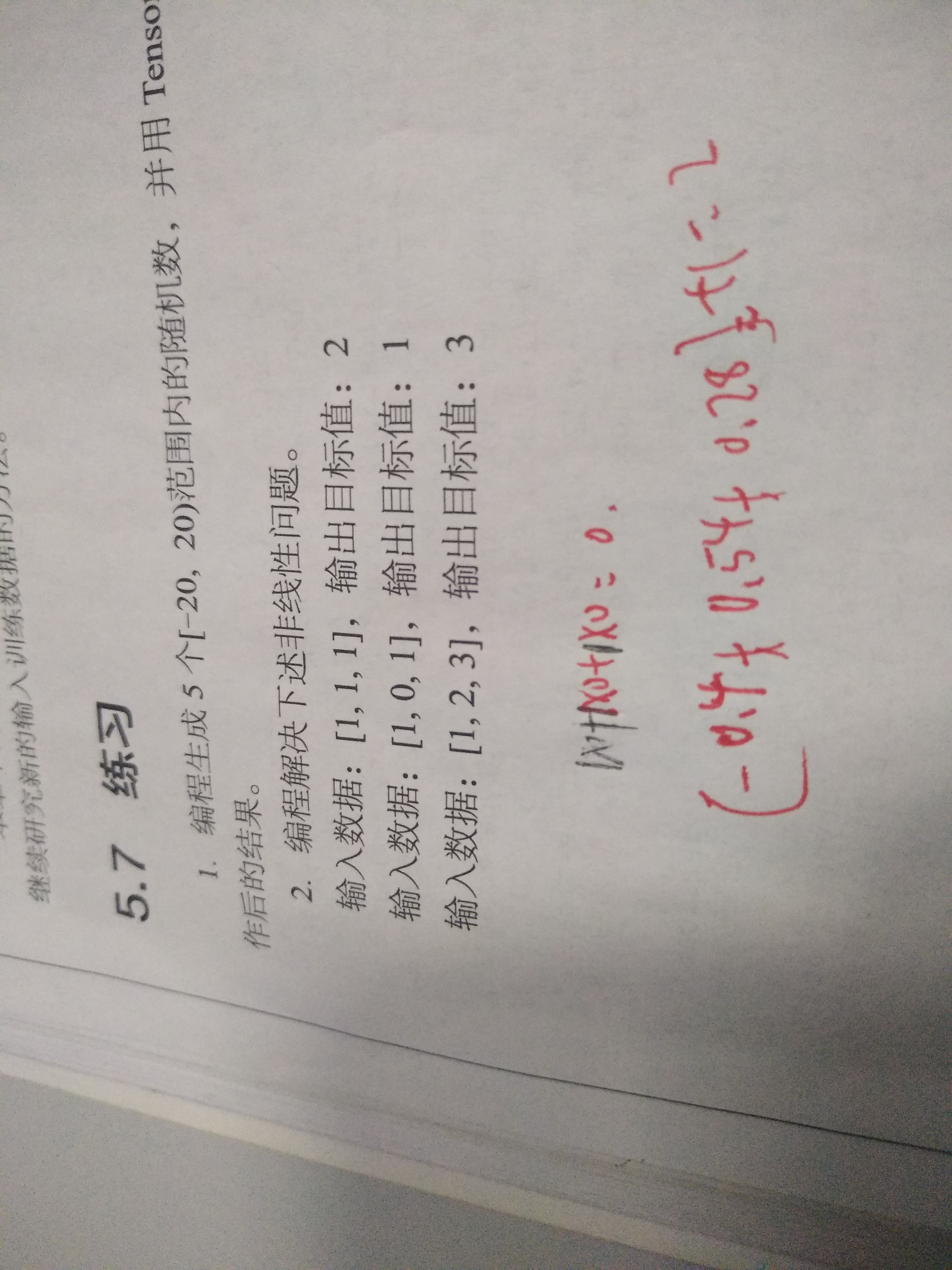•闪存
•博客
• 发言小组
• 投递新闻
• 提问博问
• 添加收藏
• 发布招聘
•文库

# 非线性问题解决

0
[待解决问题]import tensorflow as tf
import numpy as np

rowCount=3
xData = np.array([
[1,1,1],
[1,0,1],
[1,2,3],])

xTrainData = [2,1,3]
x = tf.placeholder(dtype=tf.float32)
yTrain =tf.placeholder(dtype=tf.float32)
w = tf.Variable(tf.zeros(),dtype=tf.float32)
b = tf.Variable(1,dtype=tf.float32)
n = w * x
y =tf.reduce_sum(n) + b

loss = tf.abs(y-yTrain)

optimizer = tf.train.RMSPropOptimizer(0.001)

train = optimizer.minimize(loss)

sess = tf.Session()
sess.run( tf.global_variables_initializer())
for i in range(10000):
for j in range(rowCount):
result= sess.run([train,x,w,b,yTrain,y,loss],feed_dict={x:xData[j],yTrain:xTrainData[j]})
print(result)

0@python终极者: 恕我愚钝，真的没看明白，样本太少了，如果有1000个样本，输出值只有固定几个，那就是分类问题。

您需要登录以后才能回答，未注册用户请先注册